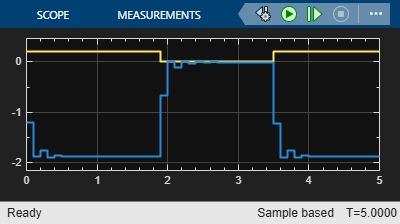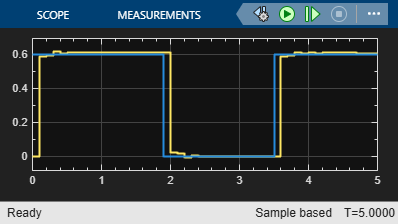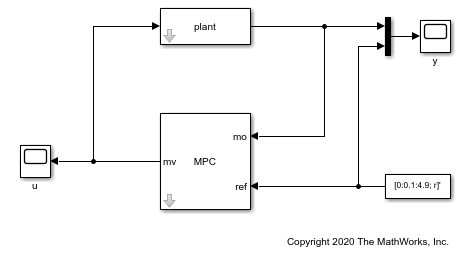# Solve a Discrete Set MPC Problem in Simulink

This example shows how to solve, using Simulink®, an MPC problem in which some manipulated variables belong to a discrete set.

### Create a Plant Model

Fix the random generator seed for reproducibility.

`rng(0);`

Create a discrete-time strictly proper plant with 4 states, two inputs and one output.

```plant = drss(4,1,2); plant.D = 0;```

Increase the control authority of the first input, to better illustrate its control contribution.

`plant.B(:,1)=plant.B(:,1)*2;`

### Design the MPC Controller

Create an MPC controller with one second sampling time, `20` steps prediction horizon and `5` steps control horizon.

`mpcobj = mpc(plant,0.1,20,5);`
```-->The "Weights.ManipulatedVariables" property is empty. Assuming default 0.00000. -->The "Weights.ManipulatedVariablesRate" property is empty. Assuming default 0.10000. -->The "Weights.OutputVariables" property is empty. Assuming default 1.00000. ```

Specify the first manipulated variable as belonging to a discrete set of seven possible values. Note that you Could also specify it as an integer using the instruction `mpcobj.MV(1).Type = 'integer';` in which case the first manipulated variable will be constrained to be an integer.

`mpcobj.MV(1).Type = [-1 -0.7 -0.3 0 0.2 0.5 1];`

Use rate limits to enforce maximum increment and decrement values for the first manipulated variable.

```mpcobj.MV(1).RateMin = -0.5; mpcobj.MV(1).RateMax = 0.5;```

Set limits on the second manipulated variable, whose default type (continuous) has not been changed.

```mpcobj.MV(2).Min = -2; mpcobj.MV(2).Max = 2;```

### Control the Plant Model in Simulink

Create an output reference signal equal to zero from steps `20` to `35` and equal to `0.6` before and after.

```r = ones(1,50)*0.6; r(20:35) = 0;```

Create a Simulink closed loop simulation using the MPC Controller block, with the `mpcobj` object passed as a parameter, to control the double integrator plant. For this example, open the pre-existing Simulink model `dcsdemo.slxc`.

`open('dcsdemo.slx')`You can now run the model by clicking Run or by using the MATLAB® command `sim`.

`sim('dcsdemo.slx')`
```-->No sample time provided for plant model. Assuming sample time = controller's sample time = 0.1. -->Assuming output disturbance added to measured output channel #1 is integrated white noise. -->The "Model.Noise" property is empty. Assuming white noise on each measured output. ```
```ans = Simulink.SimulationOutput: tout: [51x1 double] SimulationMetadata: [1x1 Simulink.SimulationMetadata] ErrorMessage: [0x0 char] ```

After the simulation, the plots of the two scopes show that the manipulated variable does not exceed the limit and the plant output tracks the reference signal after approximately half a second.